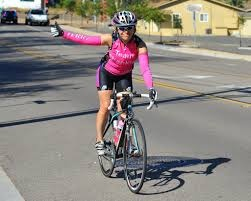# The tourist

The tourist came started from the hostel at an average speed of 5km/h. Half an hour later, the bicyclist started along the same route at a speed of 20km/h. How many minutes will a cyclist catch up and how many kilometers will he go?

Correct result:

t =  10 min
s =  3.3333 km

#### Solution:We would be pleased if you find an error in the word problem, spelling mistakes, or inaccuracies and send it to us. Thank you!Tips to related online calculators
Do you have a linear equation or system of equations and looking for its solution? Or do you have quadratic equation?
Do you want to convert velocity (speed) units?
Do you want to convert time units like minutes to seconds?

## Next similar math problems:

• Find xSolve: if 2(x-1)=14, then x= (solve an equation with one unknown)
• Simple equation 1035= 7*3*x what is x?
• Simple equationSolve for x: 3(x + 2) = x - 18
• Forest nurseryIn the forest nursery after winter, they found that 1/10 stems died out of them. For them, they land 193 new spruces. How many spruces are in the forest nursery?
• Unknown number 11That number increased by three equals three times itself?
• Simple equation 9Solve the following equation: -8y+5=-9y+9
• Negative in equation2x + 3 + 7x = – 24, what is the value of x?
• Dropped sheetsThree consecutive sheets dropped from the book. The sum of the numbers on the pages of the dropped sheets is 273. What number has the last page of the dropped sheets?
• Simple equationSolve the following simple equation: 2. (4x + 3) = 2-5. (1-x)
• SimplifySimplify the following problem and express as a decimal: 5.68-[5-(2.69+5.65-3.89) /0.5]
• GivenGiven 2x =0.125 find the value of x
• TimeageSeven times of my age is 8 less than the largest two-digit number. How old I am?
• ExpressionSolve for a specified variable: P=a+4b+3c, for a
• How oldThe student who asked how many years he answered: "After 10 years I will be twice as old than as I was four years ago. How old is student?
• Inter city busThe inter city bus leaves Suva at 10.00am and reaches Nadi at 1.00pm covering a distance of 219km. How long did it take to reach the bus Nadi?
• Average speedMichal went out of the house by car at speed 98 km/h. He came into the goal place for 270 minutes. Determine the distance between the two places.
• Distance between two citiesCar went from A to B 4h. On the way back, the car was up 15km / h faster. The return trip took 48 minutes. Shorter than the way there. Find the distance of the cities A and B.